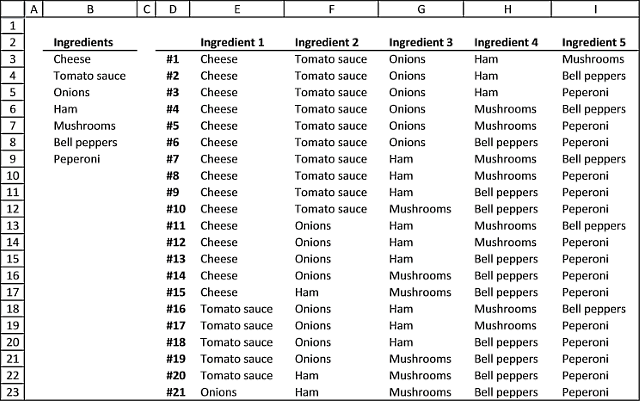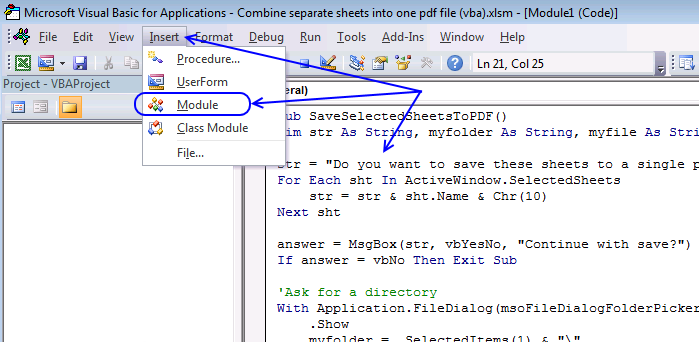Author: Oscar Cronquist Article last updated on April 16, 2021

Today I have two functions I would like to demonstrate, they calculate all possible combinations from a cell range. What is a combination? To explain combinations I must explain the difference between combinations and permutations.

Think of permutations as if the order is important and combinations as if the order is not important. If this is confusing, look at the following examples.

### Example 1 - PermutationsThink of a phone number, each digit can be between 0 to 9 or 10 different values. A five digit phone number contains 100 000 permutations (10x10x10x10x10 equals 100 000).

Now imagine a phone number to a friend or co-worker. If we rearrange the phone numbers, you could possibly call a stranger. The order is important.

Permutations with and without repetition

I discussed the difference between permutations and combinations in my last post, today I want to talk about two kinds […]

### Example 2 - CombinationsImagine you are about to buy a pizza and you can choose from five ingredients, cheese, tomato sauce, onions, ham, and mushrooms. It doesn´t matter in what order you say the ingredients. The order is not important.

A five-digit phone number has 100 000 possible permutations but five out of five pizza ingredients have only one combination. I guess only mathematicians use the word permutations, everyone else uses the word combinations even if they talk about permutations.

The following article demonstrates a problem solved with combinations, the order is not important:

Identify numbers in sum using Excel solver

Here is a useful feature I recently found googling for Excel solver examples. I have summed some random values from […]

### User defined functionsThe following User Defined Function lets you, for example, see all the combinations of pizza ingredients you can choose from if you pick 5 out of 7 ingredients.

Custom array formula in cell range E3:I23:

=Combinations(B3:B9,5)

You need to copy the VBA code below to your workbook before you try the above User Defined Function.

How to enter an array formula

1. Select cell range E3:I23
2. Click in fomula bar
3. Enter custom function
4. Press and hold CTRL + SHIFT
5. Press Enter

If you did it right the formula now begins and ends with curly brackets, like this {=array formula}. They appear automatically, don´t enter the curly brackets yourself.

A beginners guide to Excel array formulas

Array formulas allows you to do advanced calculations not possible with regular formulas.

### VBA Code

```'Dimension public variable and declare data type
Public result() As Variant

'Name User Defined Function
Function Combinations(rng As Range, n As Single)

'Save values from cell range rng to array variable rng1
rng1 = rng.Value

'Redimension array variable result
ReDim result(n - 1, 0)

'Start User Defined Function Recursive with paramters rng1, n, 1, 0
Call Recursive(rng1, n, 1, 0)

'Remove a column of values from array variable result
ReDim Preserve result(UBound(result, 1), UBound(result, 2) - 1)¨

'Transpose values in variable result and then return result to User Defined Function on worksheet
Combinations = Application.Transpose(result)

End Function
```
```'Name User Defined Function and paramters
Function Recursive(r As Variant, c As Single, d As Single, e As Single)

'Dimension variables and declare data types
Dim f As Single

'For ... Next statement
For f = d To UBound(r, 1)

'Save value in array variable r row f column 1 to array variable result row e and last column
result(e, UBound(result, 2)) = r(f, 1)

'If ... Then ... Else ... End If statement
'Check if variable in e is equal to c -1
If e = (c - 1) Then

'Add another column to array variable result
ReDim Preserve result(UBound(result, 1), UBound(result, 2) + 1)

'For ... Next statement
For g = 0 To UBound(result, 1)

'Save value in array variable result row g second last column to result row g last column
result(g, UBound(result, 2)) = result(g, UBound(result, 2) - 1)
Next g

'Continue here if e is not equal to c - 1
Else

'Start User Defined Function Recursive with parameters r, c, f + 1, e + 1
Call Recursive(r, c, f + 1, e + 1)
End If

Next f

End Function

```

These functions have a limit of 65532 rows, if you need more read this comment.

### Where to copy vba code?1. Go to VB Editor (Alt + F11)
2. Click "Insert" on the menu
3. Click "Module"
4. Paste code to code module

Tip! Did you know that Excel can calculate the number of combinations for you? Use the COMBIN function:

=COMBIN(7,5) equals 21 combinations.

Create permutations [UDF]

Chris asks: Maximum Number Allowed is 4 digit and the number is from 0 to 9. After I fill in […]

List permutations without repetition [UDF]

This blog post describes how to create permutations, repetition is NOT allowed. Permutations are items arranged in a given order meaning […]

List permutations with repetition [UDF]

This blog post demonstrates a custom function (UDF) that creates permutations. Repetition is allowed. The custom function lets you specify the […]